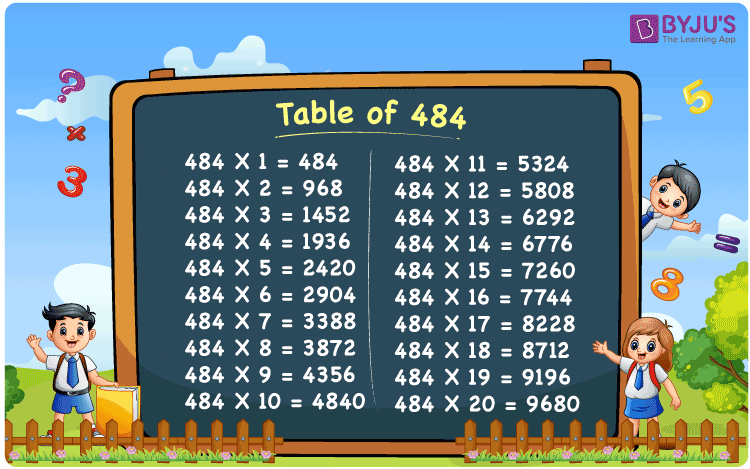Checkout JEE MAINS 2022 Question Paper Analysis : Checkout JEE MAINS 2022 Question Paper Analysis :

# Table of 484

The table of 484 is presented here up to 20 times to help students check the values of multiples of 484. The 484 times table is required for quick mental math computations that may be useful in a variety of competitive exams. Here, we have provided the multiplication table of 484 chart, which will come in handy for quick calculations and identifying other multiples of 484. You can also download a PDF of the multiplication table of 484 up to 20 times.

## Table of 484 Chart## What is the 484 Times Table?

The table of 484 is shown below in both multiplication and repeated addition form.

 484×1 = 484 484 484×2 = 968 484 + 484 = 968 484×3 = 1452 484 + 484 + 484 = 1452 484×4 = 1936 484 + 484 + 484 + 484 = 1936 484×5 = 2420 484 + 484 + 484 + 484 + 484 = 2420 484×6 = 2904 484 + 484 + 484 + 484 + 484 + 484 = 2904 484×7 = 3388 484 + 484 + 484 + 484 + 484 + 484 + 484 = 3388 484×8 = 3872 484 + 484 + 484 + 484 + 484 + 484 + 484 + 484 = 3872 484×9 = 4356 484 + 484 + 484 + 484 + 484 + 484 + 484 + 484 + 484 = 4356 484×10 = 4840 484 + 484 + 484 + 484 + 484 + 484 + 484 + 484 + 484 + 484 = 4840

## Multiplication Table of 484

The table of 484 up to 20 multiples are given below:

 484 × 1 = 484 484 × 2 = 968 484 × 3 = 1452 484 × 4 = 1936 484 × 5 = 2420 484 × 6 = 2904 484 × 7 = 3388 484 × 8 = 3872 484 × 9 = 4356 484 × 10 = 4840 484 × 11 = 5324 484 × 12 = 5808 484 × 13 = 6292 484 × 14 = 6776 484 × 15 = 7260 484 × 16 = 7744 484 × 17 = 8228 484 × 18 = 8712 484 × 19 = 9196 484 × 20 = 9680

## Solved Example on the Table of 484

Example:

Determine the value of m, if 484m = 5808.

Solution:

Given that, 484m = 5808

m = 5808/484

m = 12

Hence, the value of m is 12.

## Frequently Asked Questions on the Table of 484

### What is the table of 484?

484×1 = 484, 484×2 = 968, 484×3 = 1452, 484×4 = 1936, 484×5 = 2420, 484×6 = 2904, 484×7 = 3388, 484×8 = 3872, 484×9 = 4356, 484×10 = 4840.

### How many times does 484 equal 4840?

10 times 484 equals 4840.

### What is 484 times 15?

484 times 15 is 7260. I.e., 484 × 15 = 7260.